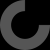# Report as inappropriate

Strange question from someone who knows very well OpenSCAD :)

``````  // Declares a user defined function add() that take 2 parameters a and b and returns their sum
// ==> This line do not call the function it just let OpenSCAD know its existence

// => Calls user defined function add() with parameters values a=1,b=1 and then gives its result as input to native function echo()

The distinction is the keyword function that begins the declaration of a new function.
A simple way to verify this is to add an echo in the function definition and see the result with and without the line echo(add(1,1));

``  function add(a,b)=let(dummy=echo("add function called with a=",a, "b=", b)) a+b;``

The same apply to modules:

``````  module test(a,b) { // Defines the module, do not call it
echo("test module called with a=",a, "b=", b);
}
test(2,3); // calls the module``````

The distinction is the keyword module that begins the declaration of a new module.

Regards